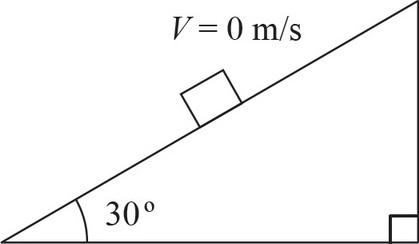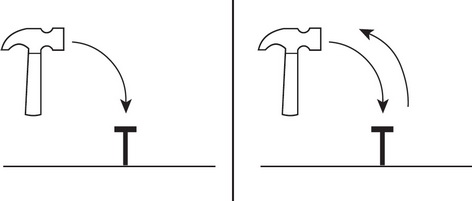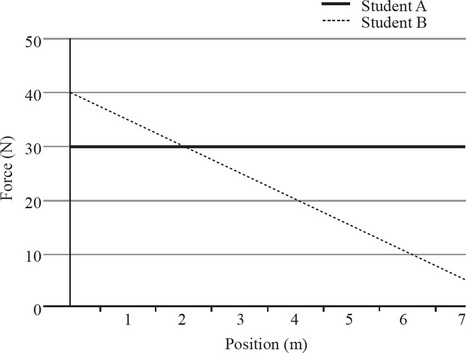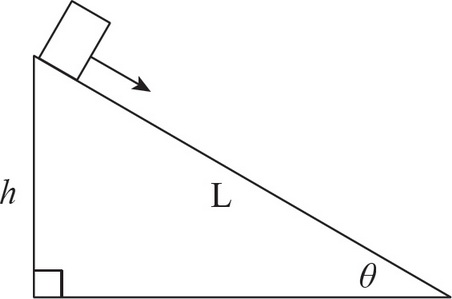# AP Physics 1 Multiple-Choice Practice Test 29

### Test Information10 questions18 minutes1. A box with a mass of 2 kg is placed on an inclined plane that makes a 30° angle with the horizontal. What must the coefficient of static friction (μs) between the box and the inclined be in order for the box to be at rest?2. A construction worker strikes a nail with a hammer twice with the same initial velocity (vi). The first time, the hammer comes to rest after hitting the nail. The second time, the hammer recoils after hitting the nail and bounces back toward the worker. Assuming the contact time in both strikes is the same, in which strike did the nail exert a greater force on the hammer?

3. A box with a mass of 5 kg is sliding across a table at a speed of 2 m/s. The coefficient of kinetic friction between the book and table is μ = 0.25. What is the minimum force that has to be applied on the box to maintain this speed?

4. Two students each push a 30 kg box across the room. Student A applies a constant force of 30 N. Student B starts with a force of 40 N but gradually reduces the force due to fatigue. Given the force-versus-position graphs of the two students, at what position have both students done the same amount of work?5. A 2000 kg truck is initially traveling with a speed of 20 m/s. The driver applies the brake and the truck slows to 10 m/s. How much work was done by the frictional force applied from the brakes?6. A box of mass m slides down a frictionless inclined plane of length L and height h. If the box is initially at rest, what is the speed of the box halfway down the inclined plane?

7. A car with a mass of 1000 kg experiences a frictional force of 3500 N while driving at a constant speed of a 15 m/s. What is the power output of the car's engine?

8. A student launched a small rocket with a mass of 50 kg into the air with an initial velocity of 10 m/s in the positive vertical direction. The student then turns on the secondary engines of the rocket to apply a constant upwards force that increases the velocity of the rocket to 15 m/s. If the force provided by the secondary engines did 4,500 J of work, how much work did the force of gravity do on the rocket?

9. A student drops a 1 kg rock off a cliff with a height of 20 m. The rock lands on the ground and comes to rest in 0.25 seconds. What was the magnitude of the average force that the rock experienced while coming to rest?

10. A plutonium atom with a mass of 244 Da (daltons) is initially at rest. The atom suddenly undergoes alpha decay, emitting an alpha particle with a mass of 4 Da with a velocity of 45 m/s east. What is the velocity of the decay product with a mass of 240 Da?